ML Aggarwal Class 6 Solutions Chapter 3 Integers Ex 3.1 for ICSE Understanding Mathematics acts as the best resource during your learning and helps you score well in your exams.

## ML Aggarwal Class 6 Solutions for ICSE Maths Chapter 3 Integers Ex 3.1

Question 1.
Write the opposite of the following:
(i) Loss of ₹ 5000
(ii) 30 km East of Delhi
(iii) 200 m above sea level
(iv) 325 BC
(v) Spending ₹ 2700
(vi) 25°C above freezing point.
Solution: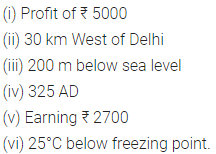Question 2.
Write each of the following using appropriate sign ‘+’ or ‘-‘:
(i) Gain of 3 kg Weight
(ii) Earning ₹ 1340
(iii) 20°C below freezing point
(iv) Loss of ₹ 470
(v) Depositing ₹ 2500 in a bank
(vi) 240 m below sea level
(vii) A jet plane flying at a height of 9320 m.
(viii) 6 m down in the basement of a building.
Solution: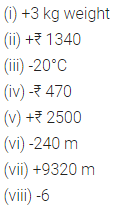Question 3.
In each of the following pairs, which number is to the right of the other on the number line?
(i) 3, 5
(ii) 0, -2
(iii) -3, -5
(iv) 2, -7
Solution: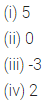Question 4.
In each of the following pairs, which number is to the left of the other on the number line?
(i) -3, 0
(ii) 2, -5
(iii) -4, -7
(iv) -10,-16
Solution: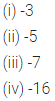Question 5.
Draw a number line and answer the following questions:
(i) Which integers lie between -9 and -2?
(ii) which is the largest among them?
(iii) Which is the smallest among them?
Solution: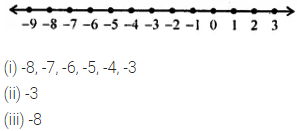Question 6.
Write four consecutive integers just greater than -9.
Solution:Question 7.
Write four consecutive integers just before -2.
Solution:Question 8.
Draw a number line and answerthe following questions:
(i) Which number will we reach if we move 6 units to the right of-1?
(ii) Which number will we reach if we move 7 units to the left of 2?
(iii) In which direction should we move to reach 3 from -3?
(iv) In which direction should we move to reach -8 from -3?
Solution: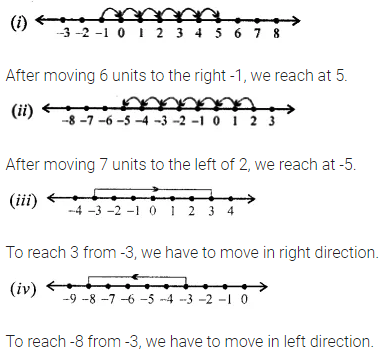Question 9.
Using the number line, write the integer which is:
(i) 5 more than -1
(ii) 5 less than -1
(iii) 7 less than 2
(iv) 3 more than -7
Solution: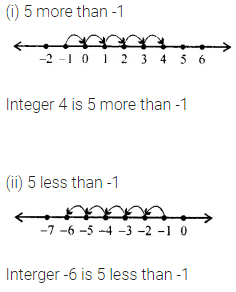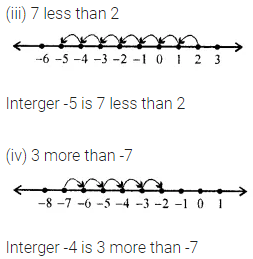Question 10.
Evaluate the following.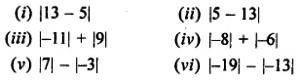Solution: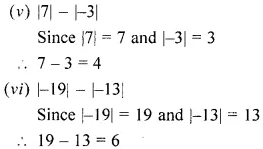Question 11.
Use the appropriate symbol < or > to fill in the following blanks:
(i) -3 ………… 7
(ii) 0 ………….. -2
(iii) -10 ………… -11
(iv) -6 …………….. -2
(v) -5 ………….. -13
(vi) -30 …………… -19
Solution:Question 12.
Arrange the following integers in ascending order:
(i) -5, 3, 0, -9, 2
(ii) -28, -33, 9, -4, -31, -2, 35
Solution: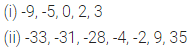Question 13.
Arrange the following integers in descending order:
(i) -31,25,-37, 43, 0,-5
(ii) -101, 95, -3, -8, 36, -7, -84
Solution:Question 14.
State whether the following statements are true (T) or false (F):
(i) 0 is the smallest positive integer.
(ii) Every negative integer is less than every natural number.
(iii) -7 is to the right of-6 on the number line,
(iv) The absolute value of an integer is always greater than the integer.
Solution: